# HSPT Math : Arithmetic

## Example Questions

### Example Question #11 : Arithmetic

Evaluate:

–3 * –7

10

–10

–21

21

4

21

Explanation:

Multiplying a negative number and another negative number makes the product positive.

### Example Question #2 : Negative Numbers

Evaluate.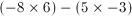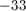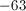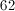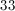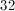Explanation:Multiplying a negative and a positive number creates a negative product: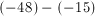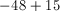### Example Question #1611 : Act Math

Solve.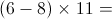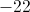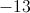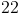Explanation: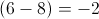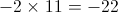A negative number multiplied by a positive number will always be negative.

### Example Question #11 : Arithmetic

Evaluate 3x3 + x2 if x = 2

28

20

14

22

20

Explanation:

When multiplying a negative number an odd number of times, the answer is negative.  When multiplying a negative number an even number of times, the answer is positive.  Order of operations also applies:  Parentheses, Exponents, Multiplication and Division, Addition and Subtraction, from left to right.  A mnemonic to remember the order of operations is “Please excuse my dear Aunt Sally.”

3(2)3 + (2)2

= 3(8) + (4)

= 24 + 4

= 20

### Example Question #12 : Arithmetic

Simplify the following expression: (–4)(2)(–1)(–3)

–16

–24

24

12

–24

Explanation:

First, we multiply –4 and 2. A negative and a positive number multiplied together give us a negative number, so (–4)(2) = –8. A negative times a negative is a positive so (–8)(–1) = 8. (8)(–3) = –24.

### Example Question #1 : Negative Numbers

What is 1 + (–1) – (–3) + 4 ?

9

1

3

6

7

7

Explanation:

You simplify the expression to be 1 – 1 + 3 + 4 = 7

### Example Question #1 : How To Multiply Negative Numbers

Multiply the following negative numbers: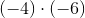.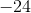Explanation:

When two negative numbers are multiplied by each other, the result is a positive number.

When a negative and a nonnegative number are multiplied with each other, the result is a negative number.

Therefore, sinceandare negative numbers, the product must be positive.

Therefore, the answer is positive.

### Example Question #12 : Negative Numbers

Evaluate: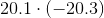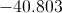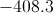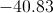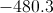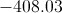Explanation:

The product of two numbers of unlike sign is the (negative) opposite of the product of their absolute values.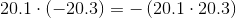There are a total of two digits to the right of the two decimal points. Therefore, the product can be calculated by multiplying 201 by 203, then placing the decimal point so that there are three digits at right.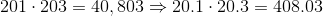Since we need to affix a negative symbol in front, the answer is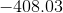### Example Question #611 : Hspt Mathematics

Solve: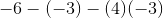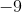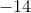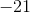Explanation:

Evaluate by order of operations, or PEMDAS. Start with multiplication of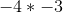. Because two negatives make a positive,  the answer for this part is.

Now we have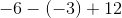and can solve from right to left. Remembering that two negatives make a positive, we now have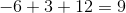The correct answer is.

### Example Question #12 : Arithmetic

Evaluate: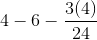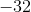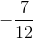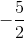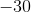Explanation:

Solve by order of operations.   Evaluate the fraction first.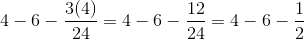Subtract the terms.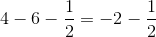In order to subrtract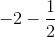we need to make common denominators.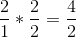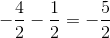### All HSPT Math Resources